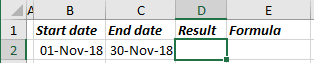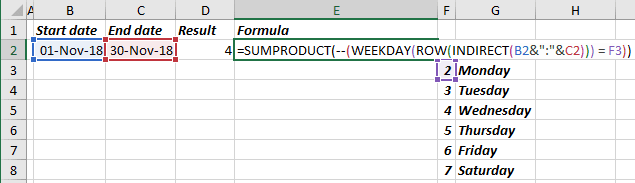# How to count days of week between two dates

Excel
From time to time, you need to count days of week between two dates or in some date range (see How to count days of week in the range of dates). Excel proposes different formulas to work with data. It is easy to create the formula you need for this task:

The formula WEEKDAY returns a number between 1 and 7 that corresponds to a particular day of the week. With default settings, 1 = Sunday, 2 = Monday, 3 = Tuesday, 4 = Wednesday, 5 = Thursday, 6 = Friday and 7 = Saturday.

For example, if it is necessary to count Mondays in November:It is easy to count the day of week in the range (see How to count days of week in the range of dates), but here you need to create the data range for the month between two dates. To do so, use ROW with INDIRECT:

date range: ROW (INDIRECT (<start date> &":"& <end date>)),

To count days of week between two dates, you need to use SUMPRODUCT formula:

= SUMPRODUCT (-- (WEEKDAY (<date range>) = 2))

or

= SUMPRODUCT (-- (WEEKDAY (<date range>) = F3))

or

= SUMPRODUCT (-- (WEEKDAY (ROW (INDIRECT (B2 &":"& C2))) = F3)):Whenever you put -- (two hyphens) in the front of the parenthesis, it changes all the values TRUE / FALSE in the array in the parenthesis to the values 1 / 0. So:

• the formula (WEEKDAY (<date range>) = 2) returns the array of TRUE and FALSE,
• (-- <array>) returns array of 1 and 0 instead of TRUE and FALSE,
• SUMPRODUCT sums all values of an array.

If you have any questions or suggestions, please feel free to ask OfficeToolTips team.